# ITC Year 3 Maths Assessment

In this assessment, students will review their comprehension of topics covered at the end of year 2 to guide their activities for assigned in year 3. May take approximately 30 minutes to complete.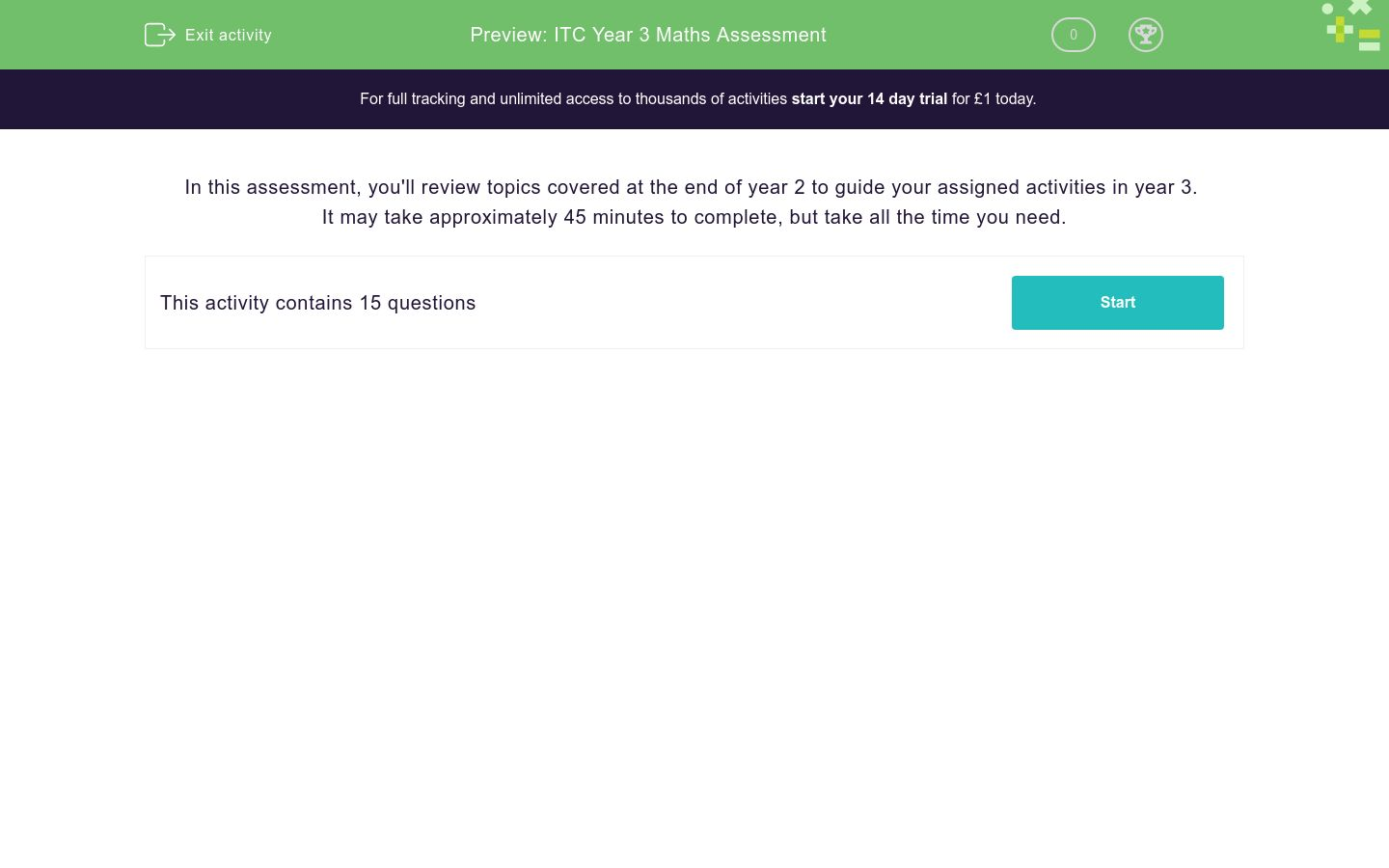Key stage:  KS 2

Curriculum topic:   Assessment

Curriculum subtopic:   Starter Assessment

Difficulty level:### QUESTION 1 of 10

In this assessment, you'll review topics covered at the end of year 2 to guide your assigned activities in year 3.

It may take approximately 45 minutes to complete, but take all the time you need.

Estimate which number the arrow points to.Put the number 21 into this tree diagram.

Where does it exit?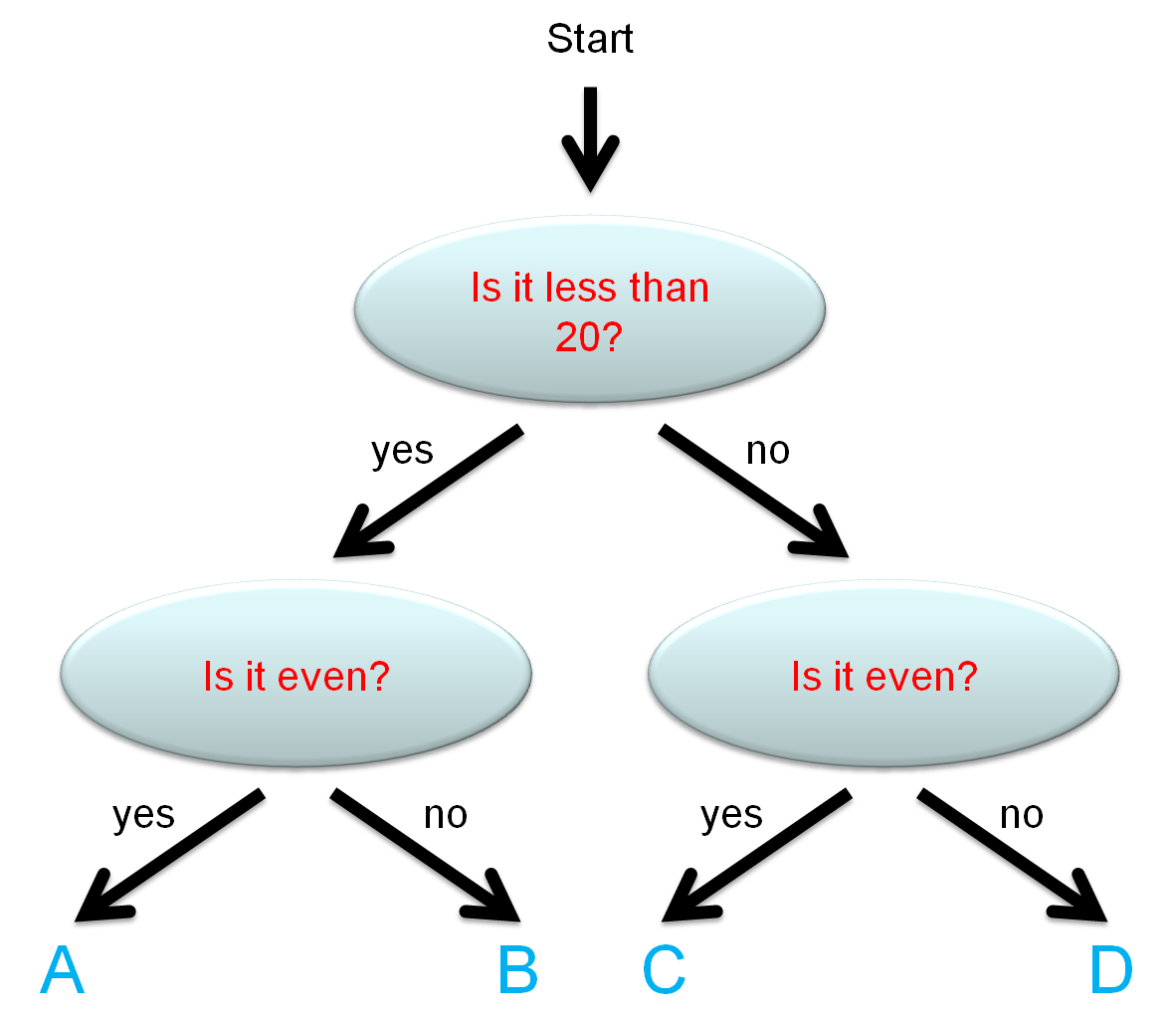A

B

C

D

Fill in the missing box.

 26 − = 15Bill builds a model rocket that is 42 cm tall.

He adds an extension that is 9 cm tall.

How tall is it now in cm?

(Just write the number.)

My jacket has got 3 pockets.

In each pocket there are 5 sweets.How many sweets are there are in total?

A piece of string is the same length as 2 pencils.How many pencils will be the same length as 4 pieces of string?Share 12 between 2

 12 ÷ 2 = ?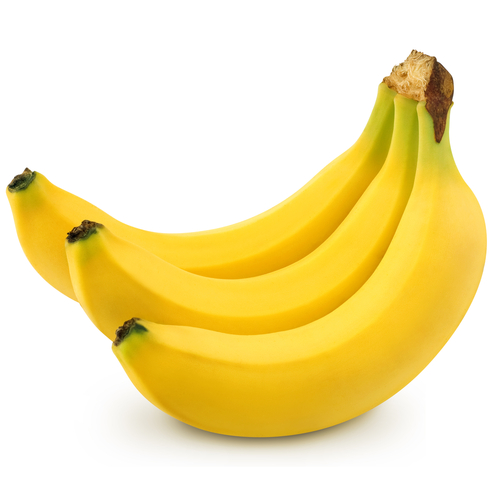A plate has 3 bananas.

A table of these plates has 21 bananas in total .

How many plates are on the table?

What fraction of these shapes are shaded red?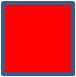3/4

1/4

1/3

1/2

Fill in the missing symbol.A cake has a mass of 250 g.

A melon has a mass of 2 kg.

mass of melonmass of cake

<

>

=

Read the scale and remember that each mark is 100 millilitres.

How much liquid is in the container in millilitres?

Just write the number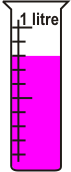Write the missing number below:

There are ______ minutes in 1 hour.

The time is

a quarter past 6.

Which clock face shows this time?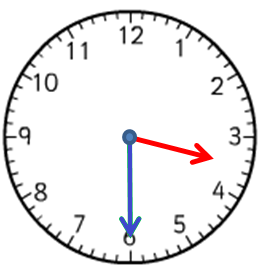A B C
A

B

C

Count the number of edges on this 3D shape.

You must count the ones you cannot see as well.How many lines of symmetry does this road sign have?• Question 1

Estimate which number the arrow points to.6
EDDIE SAYS• Question 2

Put the number 21 into this tree diagram.

Where does it exit?D
EDDIE SAYS
21 is not less than 20.
21 is not even.
• Question 3

Fill in the missing box.

 26 − = 1511
EDDIE SAYS

26 - 15 = 11

• Question 4

Bill builds a model rocket that is 42 cm tall.

He adds an extension that is 9 cm tall.

How tall is it now in cm?

(Just write the number.)

51
EDDIE SAYS

42 + 9 = 51
• Question 5

My jacket has got 3 pockets.

In each pocket there are 5 sweets.How many sweets are there are in total?

15
EDDIE SAYS

3 x 5 = 15

5 + 5 + 5 = 15

• Question 6

A piece of string is the same length as 2 pencils.How many pencils will be the same length as 4 pieces of string?8
EDDIE SAYS
4 × 2 = 8
• Question 7

Share 12 between 2

 12 ÷ 2 = ?
6
EDDIE SAYS

12 ÷ 2 = 6

2 × 6 = 12

• Question 8A plate has 3 bananas.

A table of these plates has 21 bananas in total .

How many plates are on the table?

7
EDDIE SAYS
7 × 3 = 21 bananas.
21 ÷ 3 = 7 plates.
• Question 9

What fraction of these shapes are shaded red?1/4
EDDIE SAYS
1 shape out of 4 equal shapes is shaded red.
• Question 10

Fill in the missing symbol.A cake has a mass of 250 g.

A melon has a mass of 2 kg.

mass of melonmass of cake

>
EDDIE SAYS
2000 g > 250 g
• Question 11

Read the scale and remember that each mark is 100 millilitres.

How much liquid is in the container in millilitres?

Just write the number800
EDDIE SAYS
8th mark.
• Question 12

Write the missing number below:

There are ______ minutes in 1 hour.

60
EDDIE SAYS
60 minutes = 1 hour
• Question 13

The time is

a quarter past 6.

Which clock face shows this time?A B C
A
EDDIE SAYS
The short hand is pointing to between the 6 and the 7.
The long hand is pointing to 3, which is 'a quarter past' or 15 minutes.
• Question 14

Count the number of edges on this 3D shape.

You must count the ones you cannot see as well.12
twelve
EDDIE SAYS
4 around the top.
4 around the base.
4 vertical.
• Question 15

How many lines of symmetry does this road sign have?4
EDDIE SAYS
2 through the red lines.
2 through the blue parts.
---- OR ----

Sign up for a £1 trial so you can track and measure your child's progress on this activity.

### What is EdPlace?

We're your National Curriculum aligned online education content provider helping each child succeed in English, maths and science from year 1 to GCSE. With an EdPlace account you’ll be able to track and measure progress, helping each child achieve their best. We build confidence and attainment by personalising each child’s learning at a level that suits them.

Get started